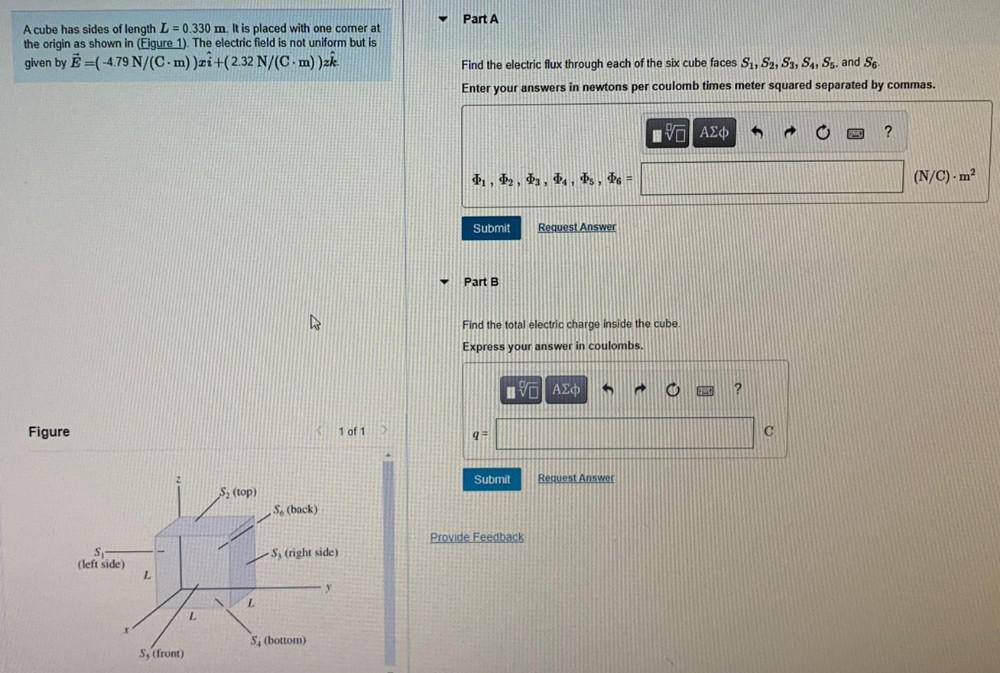Question:

# Part A A cube has sides of length L = 0.330 m. It is placed with one comer at the origin as shown in (Figure 1). The electric fiPart A A cube has sides of length L = 0.330 m. It is placed with one comer at the origin as shown in (Figure 1). The electric field is not uniform but is given by Ē=(-4.79 N/(C-m) )zi+(2.32 N/(C.m) )zł. Find the electric flux through each of the six cube faces S1, S2, S3, S4, S5, and S6 Enter your answers in newtons per coulomb times meter squared separated by commas. IV AEO ? 1, 2, 3, 4, 5, 6 = (N/C) · m² Submit Request Answer Part B Find the total electric charge inside the cube. Express your answer in coulombs. IV AXO o 3 ? Figure 1 of 1 9 C Submit Request Answer S(top) S. (back) Provide Feedback S (left side) -S, (right side) L L S. (bottom) S, (front)